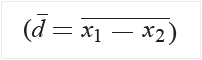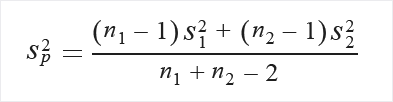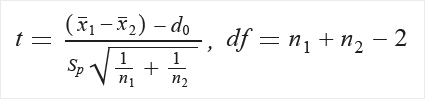# Test Hypothesis Using t-Test

Important

Support for Machine Learning Studio (classic) will end on 31 August 2024. We recommend you transition to Azure Machine Learning by that date.

Beginning 1 December 2021, you will not be able to create new Machine Learning Studio (classic) resources. Through 31 August 2024, you can continue to use the existing Machine Learning Studio (classic) resources.

ML Studio (classic) documentation is being retired and may not be updated in the future.

Compares means from two columns using a t-test

Category: Statistical Functions

Note

Applies to: Machine Learning Studio (classic) only

Similar drag-and-drop modules are available in Azure Machine Learning designer.

## Module overview

This article describes how to use the Test Hypothesis Using t-Test module in Machine Learning Studio (classic), to generate scores for three types of t-tests:

• Single sample t-test
• Paired t-test
• Unpaired t-test

In general, a t-test helps you compare whether two groups have different means. For example, suppose you are evaluating trial data for patients who received Drug A vs. patients who received Drug B, and you need to compare a recovery rate metric for both groups. The null hypothesis would assume that the recovery rate is the same in both groups, and furthermore, that the values for the recovery rate have a normal distribution in both two groups.

By using Test Hypothesis Using t-Test and providing the columns that contain the recovery rates as input, you can get scores that indicate whether the difference is meaningful, which would signify that the null hypothesis should be rejected. The test takes into account factors such as how big the difference is between the values, the size of the sample (larger is better), and how big the standard deviation is (lower is better).

By reviewing the results of the Test Hypothesis Using t-Test module, you can determine whether the null hypothesis is TRUE or FALSE, and review the confidence (P) scores from the t-test.

### How to choose a t-test

Choose a single sample t-test when these conditions apply:

• You have a single sample of scores.

• All scores are independent from each other.

• The sampling distribution of xˉ is normal.

In general, the single sample t-test is used to compare an average value to a known number.

Choose a paired t-test when these conditions apply:

• You have a matched pairs of scores. For example, you might have two different measures per person, or matched pairs of individuals (such as a husband and wife).

• Each pair of scores is independent of every other pair.

• The sampling distribution of d is normal.

A paired t-test is useful when comparing related cases. By averaging the differences between the scores of the paired cases, you can determine whether the total difference is statistically significant.

Choose an unpaired t-test when these conditions apply:

• You have two independent samples of scores. That is, there is no basis for pairing scores in sample 1 with those in sample 2.

• All scores within a sample are independent of all other scores within that sample.

• The sampling distribution of x1- x2 is normal.

• Optionally, satisfy the requirement that the variance among the groups be roughly equal.

## How to configure Test Hypothesis Using t-Test

Use a single dataset as input. The columns that you are comparing must be in the same dataset.

If you need to compare columns from different datasets, you can isolate each column to compare by using Select Columns in Dataset, and then merge them into one dataset by using Add Columns.

1. Add the Test Hypothesis Using t-Test module to your experiment.

You can find this module in the Statistical Functions category in Studio (classic).

2. Add the dataset that contains the column or columns that you want to analyze.

3. Decide which kind of t-test is appropriate for your data. See How to choose a t-test.

4. Single sample: If you are using a single sample, set these parameters:

• Null hypothesized µ: Type the value to use as the null-hypothesized mean for the sample. This specifies the expected mean value against which the sample mean will be tested.

• Target column: Use the Column Selector to choose a single numeric column for testing.

• Hypothesis type: Choose a one-tail or two-tail test. The default is a two-tailed test. This is the most common type of test, in which the expected distribution is symmetric around zero.

The One Tail GT option is for a one-tailed greater than test. This test gives more power to detect an effect in one direction, by not testing the effect in the other direction.

The One Tail LT option gives a one-tailed less than test.

• α: Specify a confidence factor. This value is used to evaluate the value of P (the first output of the module). If p is lower than the confidence factor, the null hypothesis is rejected.

5. PairedSamples: If you are comparing two samples from the same population, set these parameters:

• Null hypothesized µ: Type a value that represents the sample difference between the pair of samples.

• Target column: Use the Column Selector to choose the two numeric columns to test.

• Hypothesis type: Select either a one-tail or two-tail test. The default is a two-tailed test.

• α: Specify the confidence factor. This value is used to evaluate the value of P (the first output of the module)> If p is lower than the confidence factor, the null hypothesis is rejected.

6. UnpairedSamples: If you compare two unpaired samples, set these parameters:

• Assume equal variance: Deselect this option when the samples are from different populations.
• Null hypothesized µ1: Type the mean for the first column.
• Null hypothesized µ2: Type the mean for the second column.
• Target columns: Use the Column Selector to choose two numeric columns to test.
• Hypothesis type: Indicate whether the test is one-tail or two-tail. The default is a two-tailed test.
• α: Specify the confidence factor. This value is used to evaluate the value of P (the first output of the module)> If p is lower than the confidence factor, the null hypothesis is rejected.
7. Run the experiment.

### Results

The output of the module is a dataset containing the t-test scores, and a transformation that you can optionally save to re-apply to this or another dataset using Apply Transformation.

The dataset of scores contains these values, regardless of the type of t-test you used:

• A probability score that indicates the confidence of the null hypothesis
• A value that indicates whether the Null hypothesis should be rejected

Tip

Remember, the goal is to determine whether you can reject the null hypothesis. A score of 0 doesn't mean you should accept the null hypothesis: it means that you don't have enough data, and need further investigation.

## Technical notes

The module automatically names the output columns according to the following conventions, depending on which type of t-test was selected, and whether the result was to reject or accept the null hypothesis.

Given input columns with names {0} and {1}, the module creates the following names:

Columns SingleSampleSet PairedSamples UnpairedSamples
Output column P P_ss({0}) P_ps({0}, {1}) P_us({0}, {1})
Output column RejectH0 RejectH0_ss({0})" RejectH0_ps({0}, {1}) RejectH0_us({0}, {1})

## How Scores are computed

This module computes and uses the sample standard deviation; therefore, the equation uses `(n-1)` in the denominator.

### Computing scores for a single-sample test

Given a single sample of scores, all independent of each other, and a normal distribution, the score is calculated as follows:

1. Take the following input:

• A single column of values from the dataset
• The null hypothesis (H0) parameter μ0
• The confidence score specified by α
2. Extract the number of samples (n).

3. Calculate the mean of the sample data.

4. Calculate the standard deviation (s) of the sample data.

5. Calculate t and degrees of freedom (df):6. Extract probability P from distribution table T by using t and df.

### Computing scores for a paired t-test

Given a matched set of scores, with each pair independent of the other, and a normal distribution in each set, the score is calculated as follows:

1. Take the following input:

• Two columns of values from the dataset
• The null hypothesis (H0) parameter d0
• The confidence score specified by α
2. Extract some number of sample pairs (n).

3. Calculate the mean of differences for the sample data:4. Calculate the standard deviation of differences (sd).

5. Calculate t and the degrees of freedom (df):6. Extract probability (P) from the distribution table (T) by using t and df.

### Computing scores for an unpaired t-test

Given two independent samples of scores, with a normal distribution of values in each sample, the score is calculated as follows:

1. Take the following input:

• A dataset that contains two columns of `doubles`
• The null hypothesis (H0) parameter (d0)
• The confidence score specified by α
2. Extract a number of samples in each group, n1 and n2.

3. Calculate the means for each of the sample sets.

4. Calculate the standard deviation for each group as s1 and s2.

5. Calculate t and degrees of freedom (df):

Optionally, satisfy the requirement that the variance among the groups be roughly equal, as follows:

1. Calculate the pooled standard deviation first:2. If there is no assumption about variance equality, calculate as follows:3. Extract P from the distribution table (T) by using t and df.

### Computing the null hypothesis

The probability of the null hypothesis, designated as P, is calculated as follows:

• If P < α, set the Reject flag to True.

• If P ≥ α, set the Reject flag to False.

## Expected inputs

Name Type Description
Dataset Data Table Input dataset

## Module parameters

Name Range Type Default Description
Hypothesis type Any Hypothesis Two-tail Student's t-test null hypothesis type
Null hypothesized μ Any Float 0.0 For the single sample t-test, the null-hypothesized mean for the sample

For the paired t-test, the sample difference
Target column(s) Any ColumnSelection None Target column(s) selection pattern
Assume equal variances Any Boolean True Assume variances of two samples are equal

Applies only to unpaired samples
Null hypothesized μ1 Any Float 0.0 Null-hypothesized mean for first sample
α [0.0;1.0] Float 0.95 Confidence factor (if P is lower than the confidence factor, null hypothesis is rejected)

## Outputs

Name Type Description
P Data Table A probability score that indicates the confidence of the null hypothesis
Reject H0 Data Table Value that indicates whether the Null hypothesis should be rejected

## Exceptions

Exception Description
Error 0003 Exception occurs if one or more of inputs are null or empty.
Error 0008 Exception occurs if parameter is not in range.
Error 0017 Exception occurs if one or more specified columns have a type that is unsupported by the current module.
Error 0020 Exception occurs if the number of columns in some of the datasets passed to the module is too small.
Error 0021 Exception occurs if the number of rows in some of the datasets passed to the module is too small.
Error 0031 Exception occurs if the number of columns in column set is less than needed.
Error 0032 Exception occurs if the argument is not a number.
Error 0033 Exception occurs if the argument is infinity.

For a list of errors specific to Studio (classic) modules, see Machine Learning Error codes.

For a list of API exceptions, see Machine Learning REST API Error Codes.